# How to Read a Clock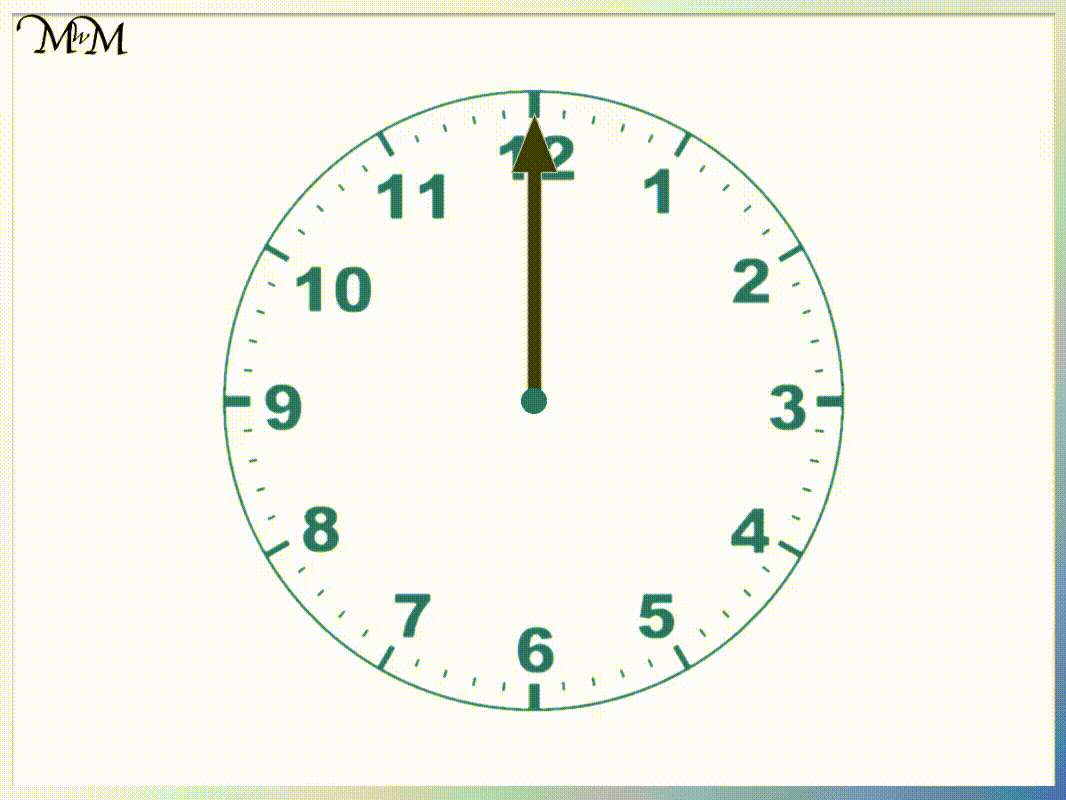• The longest hand on a clock is the minute hand.
• The marks on the outside edge of the clock face represent minutes.
• The minute hand moves from one mark to the next every minute.
• We can see that 5 minutes past is in line with the number one on the clock face.
• We can multiply each of the numbers by 5 to see how many minutes past the hour they are worth.
• Counting from each number in fives is a quicker way to see how many minutes have passed.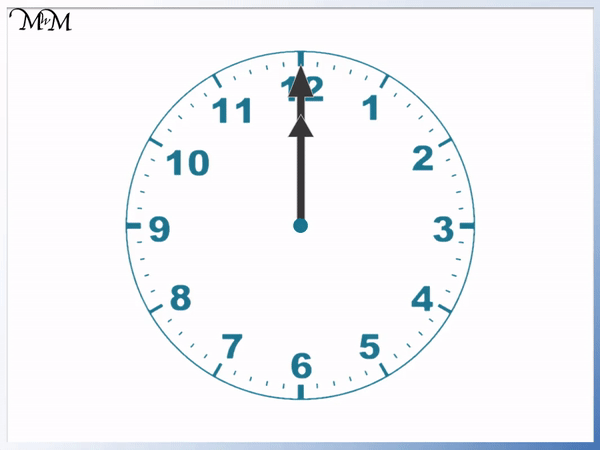• We read the minutes past the hour first using the longer minutes hand.
• We multiply the 1 on the clock by 5 to see that it means 5 minutes past.
• We count the increments from 5 minutes past to see the number of minutes past that the hand is at.
• The time is 8 minutes past the hour.
• The number that the shorter hour hand has reached tells us the hour.
• The hour hand has gone past 9 but has not yet reached 10.
• The time is 8 minutes past 9.

The number that the hour hand has reached is the hour.

The longer minutes hand tells us how many minutes past this hour we are.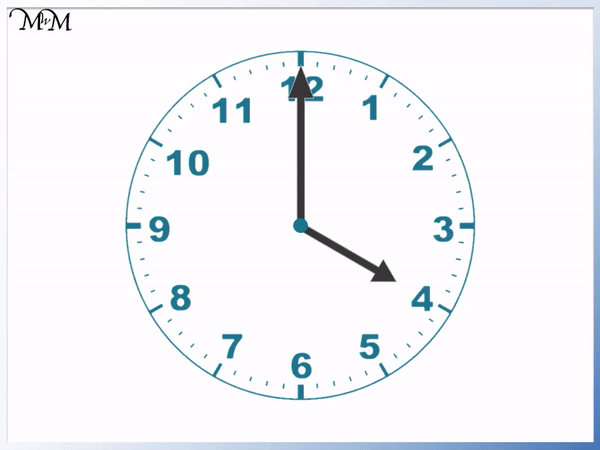• We first use the longer minutes hand to find how many minutes past the hour we have.
• The minutes hand has gone past 7 on the clock.
• 7 × 5 = 35, so the 7 is worth 35 minutes past the hour.
• We count on from 35 to see what minute the hand is exactly pointing at.
• The minute hand is pointing at 39 minutes past the hour.
• We use the shorter hour hand to find which hour.
• The hour hand has gone past 4 but not yet reached 5.
• So the time is 39 minutes past 4.# How to Tell the Time using a Clock

## Reading Minutes on a Clock

The hours on a clock are shown by the numbers around the outside. These numbers go from 1 to 12.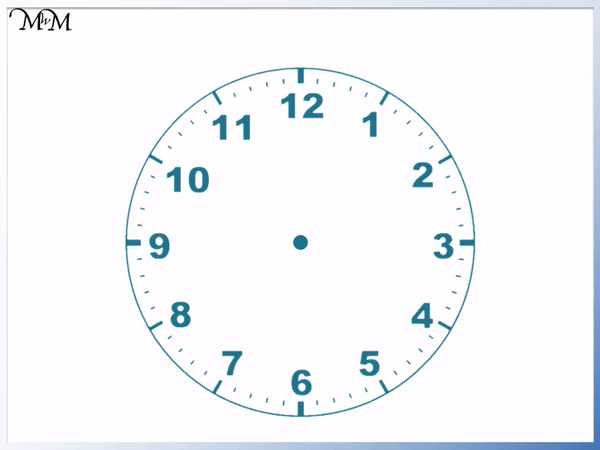A clock has two hands: the longer minute hand and the shorter hour hand.

Sometimes a clock has a 3rd hand which is the seconds hand. Since we tell the time to the nearest minute, we will not look at the seconds hand in this lesson.The shorter hour hand tells us what hour of the day it is. There are 24 hours in a day.

The longer minute hand tells us how many minutes past the hour we are.

There are 60 minutes in an hour. In each hour the hour hand moves from one number on the clock to the next.

The minute hand completes a full turn in one hour.The unnumbered marks on the outside of the clock are the minutes that have passed in each hour.

There are 60 minutes in a full hour but only 12 numbers.

60 ÷ 12 = 5 and so,

each number on the clock face is worth 5 minutes.We can see that there are 5 minute marks between each of the listed numbers.

Each number on the clock face can be multiplied by 5 to see how many minutes past the hour it represents.

## How to Tell Time on a Clock

To tell the time on a clock use the following steps:

• Look at the number written on the clock that the longer minute hand has gone past.
• Multiply this number by 5.
• Count the number of minute marks past the written number that the minute hand is pointing to.
• Add this to your multiple of 5 to get the number of minutes past the hour.
• The hour is the written number on the clock that the shorter hour hand has gone past.

For example, here is a clock face showing a time.The first step is to look at the longer minute hand to see what number written on the clock face it has gone past.

We can see that the minute hand is in between the 1 and the 2 on the clock face. The minute has has gone past the 1 but has not yet reached the 2.

We multiply 1 by 5 to get 5. This means that the 1 on the clock represents 5 minutes past the hour.

We now count on past 5 to see what minute the minute hand is pointing at.The minute hand is at 3 minutes past the 5 minute mark. We count on 6, 7, 8.

The time is 8 minutes past the hour.

To find this hour, we look at the shorter hours hand. It is in between the 9 and the 10. We can see that it has gone past the 9 but has not yet reached the 10.

The time on this clock is 8 minutes past 9.

Here is another example of reading a clock.

The first step is to look at the longer minute hand.The minute hand is pointing at a minute mark that is 2 marks past the 4.

We multiply the 4 by 5 to see what this represents in minutes.

4 × 5 = 20, so the 4 on the clock represents 20 minutes past the hour.

The minute hand is pointing at the minute mark that is 2 minutes past this.

We count on from 20: 21, 22. The minute hand is pointing at 22 minutes past the hour.The shorter hour hand is between the 2 and the 3. It has passed the 2 but not yet reached the 3.

So the time shown is 22 minutes past 2.

Here is another example of reading the time on a clock.

The minute hand is pointing at the minute mark that is 4 marks past the 7.

We multiply the 7 by 5 to see what the 7 represents in minutes.

7 × 5 = 35 and so, the 7 on the clock represents 35 minutes past the hour.

The minute hand is 4 minute marks past this. We can count on from 35: 36, 37, 38, 39.

The time is 39 minutes past the hour.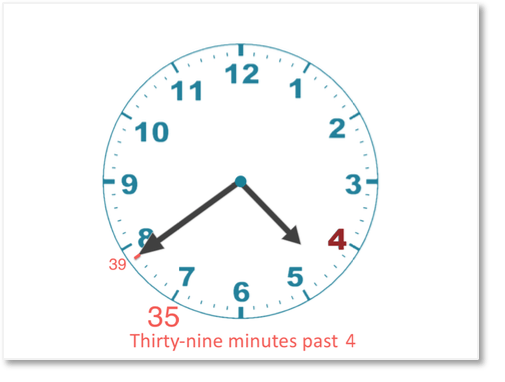The hour is shown by the shorter hour hand. The hour hand is between the 4 and the 5. It has gone past the 4.The time is 39 minutes past 4.Now try our lesson on How to Find the Coordinates of a Point where we learn how to read coordinates.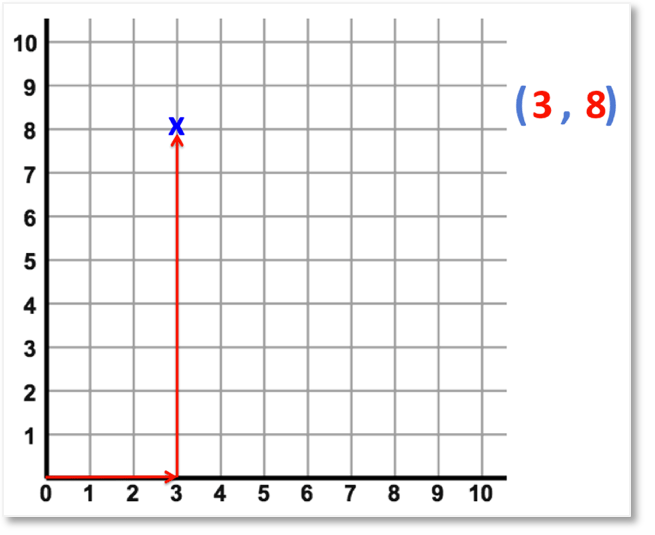error: Content is protected !!